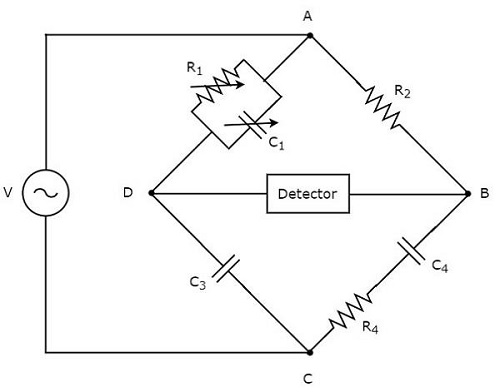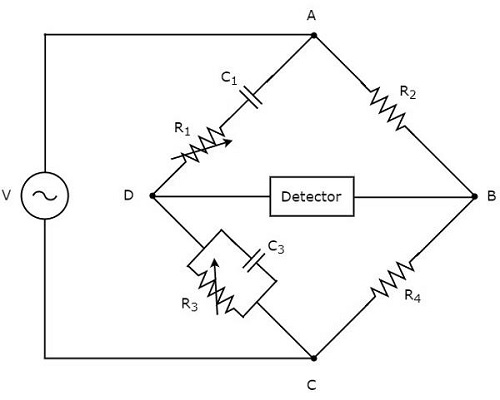# Other AC Bridges

In previous chapter, we discussed about two AC bridges which can be used to measure inductance. In this chapter, let us discuss about the following two AC bridges.

• Schering Bridge
• Wien’s Bridge

These two bridges can be used to measure capacitance and frequency respectively.

## Schering Bridge

Schering bridge is an AC bridge having four arms, which are connected in the form of a rhombus or square shape, whose one arm consists of a single resistor, one arm consists of a series combination of resistor and capacitor, one arm consists of a single capacitor & the other arm consists of a parallel combination of resistor and capacitor.

The AC detector and AC voltage source are also used to find the value of unknown impedance, hence one of them is placed in one diagonal of Schering bridge and the other one is placed in other diagonal of Schering bridge.

Schering bridge is used to measure the value of capacitance. The circuit diagram of Schering bridge is shown in the below figure.In above circuit, the arms AB, BC, CD and DA together form a rhombus or square shape. The arm AB consists of a resistor, $R_{2}$. The arm BC consists of a series combination of resistor, $R_{4}$ and capacitor, $C_{4}$. The arm CD consists of a capacitor, $C_{3}$. The arm DA consists of a parallel combination of resistor, $R_{1}$ and capacitor, $C_{1}$.

Let, $Z_{1}$, $Z_{2}$,$Z_{3}$ and $Z_{4}$ are the impedances of arms DA, AB, CD and BC respectively. The values of these impedances will be

$Z_{1}=\frac{R_{1}\left ( \frac{1}{j \omega C_{1}} \right )}{R_{1}+\frac{1}{j \omega C_{1}}}$

$\Rightarrow Z_{1}=\frac{R_{1}}{1+j \omega R_{1}C_{1}}$

$Z_{2}=R_{2}$

$Z_{3}=\frac{1}{j \omega C_{3}}$

$Z_{4}=R_{4}+\frac{1}{j \omega C_{4}}$

$\Rightarrow Z_{4}=\frac{1+j \omega R_{4}C_{4}}{j \omega C_{4}}$

Substitute these impedance values in the following balancing condition of AC bridge.

$$Z_{4}=\frac{Z_{2}Z_{3}}{Z_{1}}$$

$$\frac{1+j \omega R_{4}C_{4}}{j \omega C_{4}}=\frac{R_{2}\left (\frac{1}{j \omega C_{3}} \right )}{\frac{R_{1}}{1+j \omega R_{1}C_{1}}}$$

$\Rightarrow \frac{1+j \omega R_{4}C_{4}}{j \omega C_{4}}=\frac{R_{2}\left ( 1+j \omega R_{1}C_{1} \right )}{j \omega R_{1}C_{3}}$

$\Rightarrow \frac{1+j \omega R_{4}C_{4}}{C_{4}}=\frac{R_{2}\left ( 1+j \omega R_{1}C_{1} \right )}{R_{1}C_{3}}$

$\Rightarrow \frac{1}{C_{4}}+j \omega R_{4}=\frac{R_{2}}{R_{1}C_{3}}+\frac{j\omega C_{1}R_{2}}{C_{3}}$

By comparing the respective real and imaginary terms of above equation, we will get

$C_{4}=\frac{R_{1}C_{3}}{R_{2}}$Equation 1

$R_{4}=\frac{C_{1}R_{2}}{C_{3}}$Equation 2

By substituting the values of $R_{1}, R_{2}$ and $C_{3}$ in Equation 1, we will get the value of capacitor, $C_{4}$. Similarly, by substituting the values of $R_{2}, C_{1}$ and $C_{3}$ in Equation 2, we will get the value of resistor, $R_{4}$.

The advantage of Schering bridge is that both the values of resistor, $R_{4}$ and capacitor, $C_{4}$ are independent of the value of frequency.

## Wien’s Bridge

Wien’s bridge is an AC bridge having four arms, which are connected in the form of a rhombus or square shape. Amongtwo arms consist of a single resistor, one arm consists of a parallel combination of resistor and capacitor & the other arm consists of a series combination of resistor and capacitor.

The AC detector and AC voltage source are also required in order to find the value of frequency. Hence, one of these two are placed in one diagonal of Wien’s bridge and the other one is placed in other diagonal of Wien’s bridge.

The circuit diagram of Wien’s bridge is shown in the below figure.In above circuit, the arms AB, BC, CD and DA together form a rhombus or square shape. The arms, AB and BC consist of resistors, $R_{2}$ and $R_{4}$ respectively. The arm, CD consists of a parallel combination of resistor, $R_{3}$ and capacitor, $C_{3}$. The arm, DA consists of a series combination of resistor, $R_{1}$ and capacitor, $C_{1}$.

Let, $Z_{1}, Z_{2}, Z_{3}$ and $Z_{4}$ are the impedances of arms DA, AB, CD and BC respectively. The values of these impedances will be

$$Z_{1}=R_{1}+\frac{1}{j \omega C_{1}}$$

$$\Rightarrow Z_{1}=\frac{1+j \omega R_{1}C_{1}}{j \omega C_{1}}$$

$Z_{2}=R_{2}$

$$Z_{3}=\frac{R_{3}\left (\frac{1}{j \omega C_{3}} \right )}{R_{3}+\frac{1}{j \omega C_{3}}}$$

$$\Rightarrow Z_{3}= \frac{R_{3}}{1+j \omega R_{3}C_{3}}$$

$Z_{4}=R_{4}$

Substitute these impedance values in the following balancing condition of AC bridge.

$$Z_{1}Z_{4}=Z_{2}Z_{3}$$

$$\left (\frac{1+j \omega R_{1}C_{1}}{j \omega C_{1}} \right )R_{4}=R_{2}\left (\frac{R_{3}}{1+j \omega R_{3}C_{3}} \right )$$

$\Rightarrow \left (1+j \omega R_{1}C_{1}\right )\left (1+j \omega R_{3}C_{3}\right )R_{4}=j \omega C_{1}R_{2}R_{3}$

$\Rightarrow \left (1+j \omega R_{3}C_{3}+j \omega R_{1}C_{1}-\omega^{2}R_{1}R_{3}C_{1}C_{3}\right )R_{4}=j \omega C_{1}R_{2}R_{3}$

$\Rightarrow R_{4}\left ( \omega^{2}R_{1}R_{3}C_{1}C_{3} \right )+j \omega R_{4}\left (R_{3}C_{3}+R_{1}C_{1} \right )=j \omega C_{1}R_{2}R_{3}$

Equate the respective real terms of above equation.

$$R_{4}\left (1- \omega^{2}R_{1}R_{3}C_{1}C_{3} \right )=0$$

$\Rightarrow 1- \omega^{2}R_{1}R_{3}C_{1}C_{3} =0$

$\Rightarrow 1= \omega^{2}R_{1}R_{3}C_{1}C_{3}$

$\omega = \frac{1}{\sqrt{R_{1}R_{3}C_{1}C_{3}}}$

Substitute, $\omega = 2 \pi f$ in above equation.

$$\Rightarrow 2 \pi f= \frac{1}{\sqrt{R_{1}R_{3}C_{1}C_{3}}}$$

$\Rightarrow f= \frac{1}{2 \pi\sqrt{R_{1}R_{3}C_{1}C_{3}}}$

We can find the value of frequency, $f$ of AC voltage source by substituting the values of $R_{1}, R_{3}, C_{1}$ and $C_{3}$ in above equation.

If $R_{1}=R_{3}=R$ and $C_{1}=C_{3}=C$, then we can find the value of frequency, $f$ of AC voltage source by using the following formula.

$$f=\frac{1}{2\pi RC}$$

The Wein’s bridge is mainly used for finding the frequency value of AF range.